# Construction Of Similar Triangles

Construction of Similar Triangles is one of the important concepts of class 10 maths, and this concept has some weightage for the students in their exams. In this article, you will learn how to construct a triangle similar to the given triangle. Before we understand the construction of a similar triangle, let us know what similar triangles are?

### Similar Triangles

If two triangles ∆ABC and ∆PQR are said to be similar triangles, then the following two conditions must be satisfied:

(i) The corresponding angles of the two triangles are equal.

i.e. ∠A = ∠P, ∠B = ∠Q, ∠C = ∠R

(ii) Corresponding sides are in a ratio or proportion.

$$\begin{array}{l} \frac {AB}{PQ} \end{array}$$
=
$$\begin{array}{l} \frac {BC}{QR} \end{array}$$
=
$$\begin{array}{l} \frac {AC}{PR} \end{array}$$

Thus, the similarity of ∆ABC and ∆PQR can be written as ∆ABC ~ ∆PQR and it is read as “∆ABC is similar to ∆PQR”.

Example:

Consider the two triangles namely ∆ABC and ∆PQR given in the figure such that ∆ABC~∆PQR. What is the length of the side PR if AB = 6 cm, AC = 8 cm and PQ = 3 cm?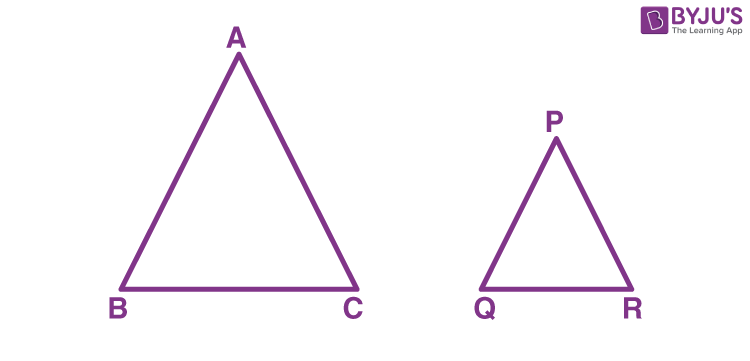Since, ∆ABC~∆PQR

$$\begin{array}{l} \frac {AB}{PQ} \end{array}$$
=
$$\begin{array}{l} \frac {AC}{PR} \end{array}$$

$$\begin{array}{l} \frac {6}{3} \end{array}$$
=
$$\begin{array}{l} \frac {8}{PR} \end{array}$$

PR =

$$\begin{array}{l} \frac {8~×~3}{6} \end{array}$$
= 4 cm

Let’s see how to construct similar triangles that come in constructions class 10.

## Construction of Similar Triangles Using Scale Factor

The construction of a similar triangle involves two different cases in which the triangle to be constructed is bigger (or larger), whereas, in the other, it is smaller than the given triangle. Also, the scale factor determines the ratio of the triangle sides to be constructed with the corresponding sides of the given triangle.

Case 1: In this case, the scale factor is greater than 1 and thus the constructed similar triangle is bigger than the given triangle.

Case 2: In this case, the scale factor is less than 1 and thus the constructed similar triangle is smaller than the given triangle.

These two cases are explained below, along with the required steps.

### Case 1

Construct a triangle similar to the given triangle ABC with a scale factor of 5/3.

Here, scale factor 5/3 means, the new triangle will have side lengths 5/3 times the corresponding side lengths.

Steps of Construction:

Step 1: Construct a triangle ABC as given below: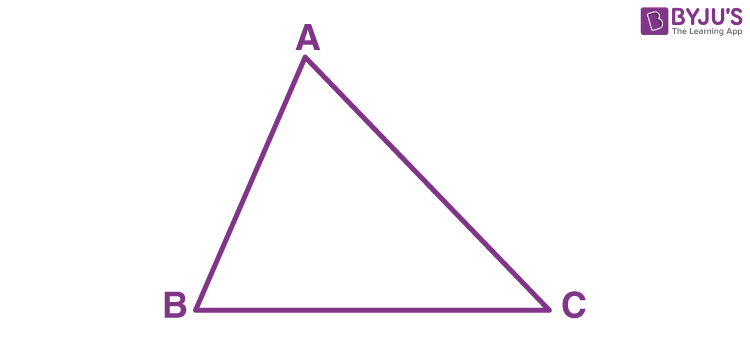Step 2: Draw a ray BX making an acute acute with the base BC and mark 5 points B1, B2, B3, B4, B5 on BX such that BB1 = B1B2 = B2B3 = B3B4 = B4B5.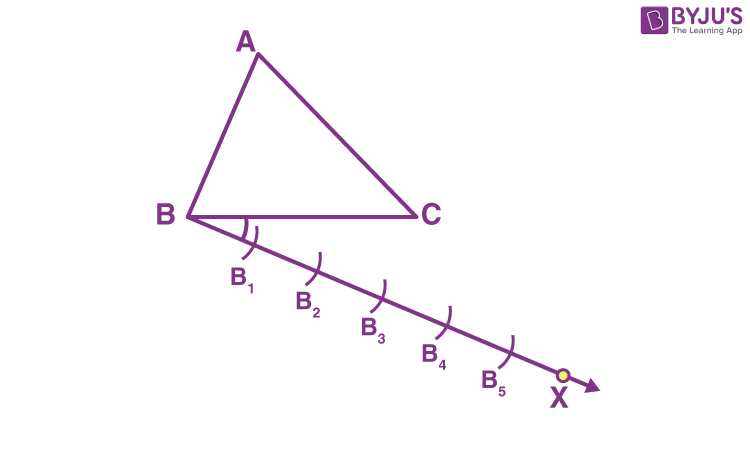Step 3: Join B3C and draw a line B5C’ such that B3C is parallel to B5C’, where C’ lies on the produced BC.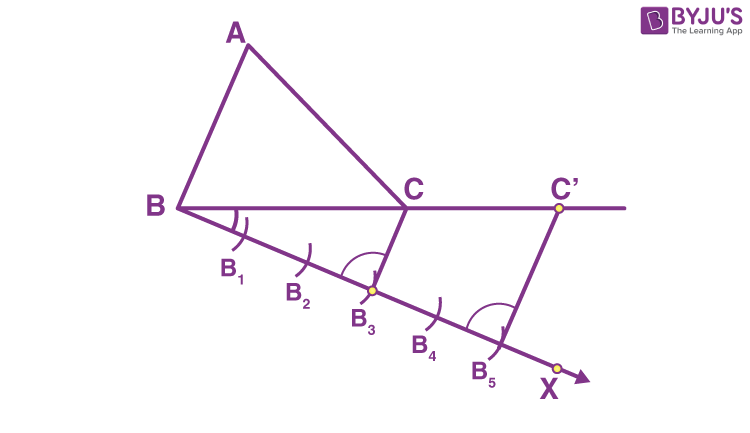Step 4: Now draw another line parallel to AC at C’ such that it meets the produced BA at A’.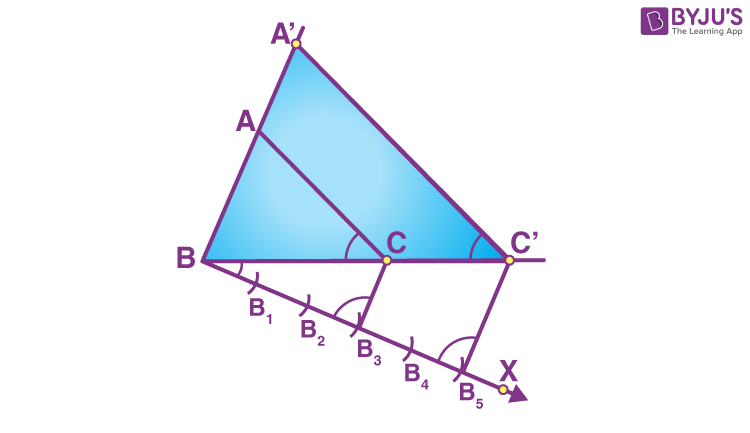Hence, ΔA’BC’ is the required triangle similar to the ΔABC.

### Case 2

Construct a triangle that is similar to ∆ABC with a scale factor of 3/5.

Here, scale factor 3/5 means, the new triangle will have side lengths 3/5 times the corresponding side lengths.

Consider the given triangle ABC: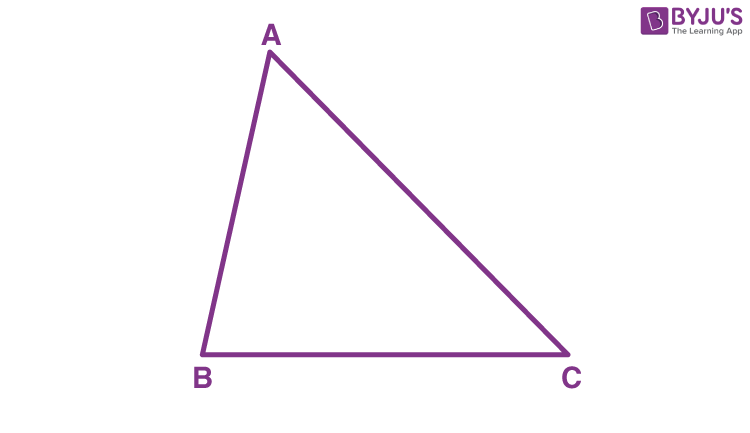Steps of Construction:

Step 1: Draw a ray BX which makes an acute angle with BC on the opposite side of vertex A.

Step 2: Locate 5 points on the ray BX and mark them as B1, B2, B3, B4, B5 on BX such that BB1 = B1B2 = B2B3 = B3B4 = B4B5.

Step 3: Join B5C

Step 4: Draw a line parallel to B5C through B3 (since 3 is the smallest among 3 and 5) and mark C’ where it intersects with BC.

Step 5: Draw a line through the point C’ parallel to AC and mark A’ where it intersects AB.

Thus, A’BC’ is the required triangle.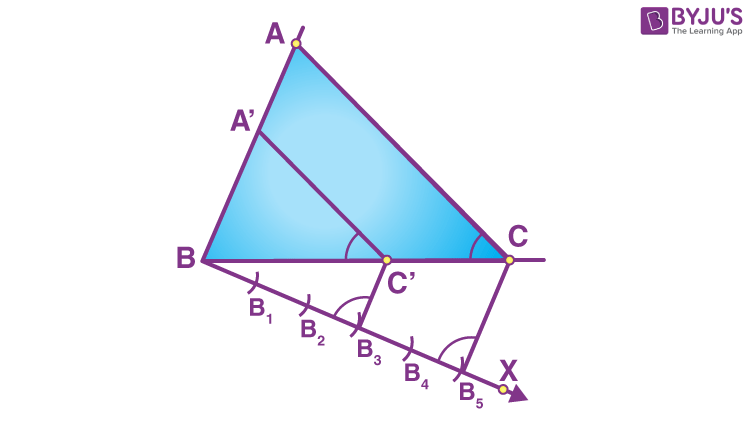How can we verify that ∆ABC ~ ∆A’BC’?

$$\begin{array}{l} \frac {BC’}{C’C}\end{array}$$
=
$$\begin{array}{l} \frac 32 \end{array}$$
[By construction]

Therefore,

$$\begin{array}{l} \frac {BC}{BC’}\end{array}$$
=
$$\begin{array}{l} \frac {BC’~+~C’C}{BC’}\end{array}$$
=
$$\begin{array}{l} 1~+~\frac 23 \end{array}$$
=
$$\begin{array}{l} \frac 53 \end{array}$$

That gives,

$$\begin{array}{l} \frac {BC’}{BC}\end{array}$$
=
$$\begin{array}{l} \frac 35 \end{array}$$

Here, A’C’ is parallel to AC.

∠ A’C’B = ∠ACB [corresponding angles]

∠ABC = ∠A’BC’ [common angle]

Therefore,

∆ABC ~ ∆A’BC’.

## Video Lesson on BPT and Similar Triangles### Solved Problem

Let us understand this construction with the help of an example.

Example:

Consider ∆ABC where BC = 6 cm, ∠B = 40°and ∠C = 60°. Draw a triangle similar to ∆ABC with a scale factor 2.

Solution: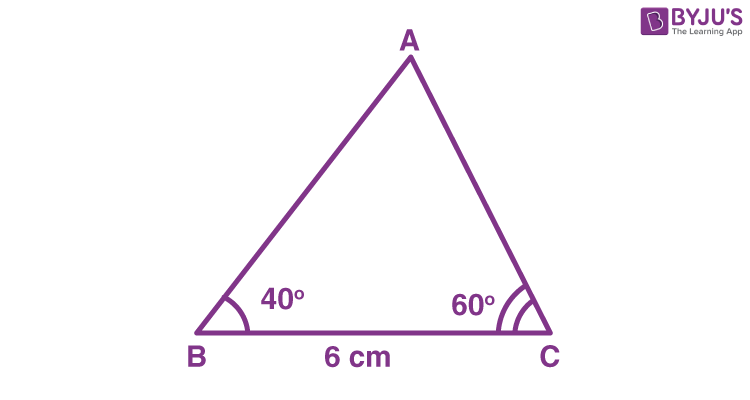Here, a scale factor of 2 means that the sides of the new triangle which is similar to ∆ABC are twice the sides of ∆ABC.

Let ∆PQR be the new triangle.

QR = 2 × 6 = 12 cm [scale factor is 2]

∠B = ∠Q = 40°and ∠C = ∠R = 60°.

Steps of construction:

• Draw QR of length 12 cm
• Draw a line through Q which makes an angle of 40° from QR.
• Draw a line through R which makes an angle of 60° from QR.
• Mark the intersection point of two lines above QR as P. Thus, ∆PQR is the required triangle as given below.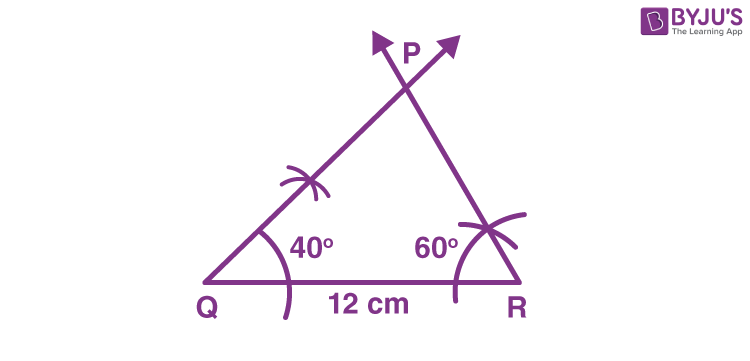Now, suppose the scale factor is a fraction, like

$$\begin{array}{l} \frac 54 \end{array}$$
,
$$\begin{array}{l} \frac 78 \end{array}$$
etc and we don’t know the length of the sides, then we won’t be able to construct similar triangles precisely.

Note:

From the above constructions, it is clear that, if the scale factor is equal to less than 1, then the triangle to be constructed similar to the given triangle will be smaller than the original triangle otherwise it will be larger than the given triangle.

### Practice Questions

1. Construct a triangle of sides 4 cm, 5 cm and 6 cm and then a triangle similar to it whose sides are 2/3 of the corresponding sides of the first triangle.
2. Construct an isosceles triangle whose base is 8 cm and altitude 4 cm and then another triangle whose sides are 1½ times the corresponding sides of the isosceles triangle.
3. Draw a triangle ABC in which AB = 4 cm, BC = 6 cm and AC = 9 cm. Construct a triangle similar to ∆ABC with a scale factor of 3/2. Justify the construction. Are the two triangles congruent? Note that all the three angles and two sides of the two triangles are equal.